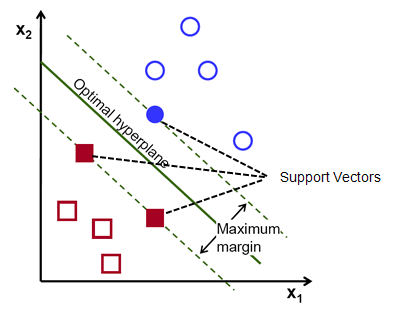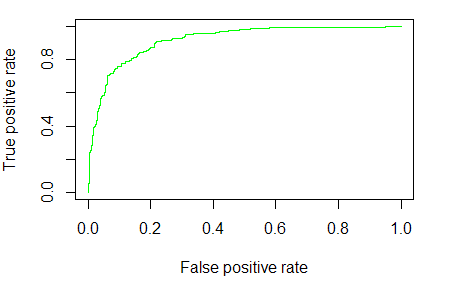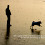# Support Vector Machine Simplified using R

This tutorial describes theory and practical application of Support Vector Machines (SVM) with R code. It's a popular supervised learning algorithm (i.e. classify or predict target variable). It works both for classification and regression problems. It's one of the sought-after machine learning algorithm that is widely used in data science competitions.SVM with R

What is Support Vector Machine?

The main idea of support vector machine is to find the optimal hyperplane (line in 2D, plane in 3D and hyperplane in more than 3 dimensions) which maximizes the margin between two classes. In this case, two classes are red and blue balls. In layman's term, it is finding the optimal separating boundary to separate two classes (events and non-events).Support Vector Machine (SVM)
Support Vectors are observations that supports hyperplane on either sides. In the image above, filled red and blue boxes and circles are support vectors.

Why Hyperplane?

Hyperplane is just a line in 2D and plane in 3D. In higher dimensions (more than 3D), it's called hyperplane. SVM help us to find a hyperplane (or separating boundary) that can separate two classes (red and blue dots).

What is Margin?

It is the distance between the hyperplane and the closest data point. If we double it, it would be equal to the margin.
Objective : Maximize the margin between two categories

How to find the optimal hyperplane?

In your dataset, select two hyperplanes which separate the data with no points between them and maximize the distance between these two hyperplanes. The distance here is 'margin'.

How to treat Non-linear Separable Data?
Imagine a case - if there is no straight line (or hyperplane) which can separate two classes? In the image shown below, there is a circle in 2D with red and blue data points all over it such that adjacent data points are of different colors.SVM : Nonlinear Separable Data

SVM handles the above case by using a kernel function to handle non-linear separable data. It is explained in the next section.

What is Kernel?

In simple words, it is a method to make SVM run in case of non-linear separable data points. The kernel function transforms the data into a higher dimensional feature space to make it possible to perform the linear separation. See the image below -SVM Kernel
In this case, we have transformed our data into a 3 dimensional space. Now data points are separated by a simple plane. It is possible by kernel function. We can say a nonlinear function is learned by a support vector (linear learning) machine in a high-dimensional feature space.

Different Kernels

1. linear: u'*v
2. polynomial: (gamma*u'*v + coef0)^degree
3. radial basis (RBF) : exp(-gamma*|u-v|^2)
4. sigmoid : tanh(gamma*u'*v + coef0)

RBF is generally the most popular one.

How SVM works?
1. Choose an optimal hyperplane which maximize margin
2. Applies penalty for misclassification (cost 'c' tuning parameter).
3. If non-linearly separable data points, transform data to high dimensional space where it is easier to classify with linear decision surfaces (Kernel trick)

1. SVM performs well in case of non-linear separable data using kernel trick.
2. It works well in high dimensional space (i.e. large number of predictors)
3. It works well in case of text or image classification.
4. It does not suffer multicollinearity problem

1. It takes a lot of time on large sized data sets
2. It does not directly return probability estimates.
3. The linear kernel is almost similar to logistic regression in case of linear separable data.

Multi-Category Classes and SVM
Multi-category classes can be split into multiple one-versus-one or one-versus-rest binary classes.

Support Vector Machine - Regression

Yes, Support Vector Machine can also be used for regression problem wherein dependent or target variable is continuous.

The goal of SVM regression is same as classification problem i.e. to find maximum margin. Here, it means minimize error. In the case of regression, a margin of tolerance (epsilon) is set in approximation to the SVM. The primary goal is to minimize error, individualizing the hyperplane which maximizes the margin, keeping in mind that part of the error is tolerated.

SVM - Standardization

All kernel methods are based on distance. Hence, it is required to scale our variables. If we do not standardize our variables to comparable ranges, the variable with the largest range will completely dominate in the computation of the kernel matrix. For example, we have two variables - X1 and X2. Values of variable X1 lies between 0 and 100 whereas values of X2 lies in range of 100 and 10000. In this case, variable X2 would dominate variable X1. It is recommended to standardize each variables to the range [-1.1] or [0,1]. The z-score and min-max are the two popular methods to standardize variables.

Another reason of standardization is to avoid numerical difficulties during computation. Because kernel values usually depend on the inner products of feature vectors, e.g. the linear kernel and the polynomial kernel, large attribute values might cause numerical problems.

Tuning Parameters of SVM

If Linear Kernel SVM

There is only one parameter in linear kernel - Cost (C). It implies misclassification cost on training data.
1. A large C gives you low bias and high variance. Low bias because you penalize the cost of misclassification a lot. Large C makes the cost of misclassification high, thus forcing the algorithm to explain the input data stricter and potentially overfit.
2. A small C gives you higher bias and lower variance. Small C makes the cost of misclassification low, thus allowing more of them for the sake of wider "cushion"
The goal is to find the balance between "not too strict" and "not too loose". Cross-validation and resampling, along with grid search, are good ways to finding the best C.

Two parameters for fine tuning in radial kernel - Cost and Gamma

The parameter cost is already explained above (See the 'Linear Kernel' section).

Gamma explains how far the influence of a single training example reaches. When gamma is very small, the model is too constrained and cannot capture the complexity or “shape” of the data.

R : Grid Search
cost=10^(-1:2), gamma=c(.5, 1, 2)
Polynomial kernel Tuning Parameters:
1. degree (Polynomial Degree)
2. scale (Scale)
3. C (Cost)

Support Vector Regression Tuning Parameters:
1. Epsilon (e)
2. Cost (c)
epsilon = seq(0, 0.9, 0.1),  cost = 2^(2:8)

R Code : Support Vector Machine (SVM)

Make sure you have the following packages already installed. If not, install them by using install.packages() function.
library(caret)
library(kernlab)
library(ROCR)

In this case, we are using segmentation data which is already loaded in caret package.
data(segmentationData)

Data Exploration
#Number of rows and columns
dim(segmentationData)
 2019   61

#Distribution of Target Variable
table(segmentationData\$Class)
PS   WS
1300  719

table(segmentationData\$Class) / length(segmentationData\$Class)
PS        WS
0.6438831 0.3561169

Split Data into Training and Validation
Index <- createDataPartition(segmentationData\$Class,p=.7,list=FALSE)
svm.train <- segmentationData[Index,]
svm.validate  <- segmentationData[-Index,]
trainX <-svm.train[,4:61]

Build SVM model in R

# Setup for cross validation
set.seed(123)
ctrl <- trainControl(method="cv",
number = 2,
summaryFunction=twoClassSummary,
classProbs=TRUE)

# Grid search to fine tune SVM
grid <- expand.grid(sigma = c(.01, .015, 0.2),
C = c(0.75, 0.9, 1, 1.1, 1.25)
)

#Train SVM
svm.tune <- train(x=trainX,
y= svm.train\$Class,
metric="ROC",
tuneGrid = grid,
trControl=ctrl)

svm.tune

# Predict Target Label
valX <-svm.validate[,4:61]
pred <- predict(svm.tune, valX, type="prob")

# Model Performance Statistics
pred_val <-prediction(pred[,2], svm.validate\$Class)

# Calculating Area under Curve
perf_val <- performance(pred_val,"auc")
perf_val

# Calculating True Positive and False Positive Rate
perf_val <- performance(pred_val, "tpr", "fpr")

# Plot the ROC curve
plot(perf_val, col = "green", lwd = 1.5)

#Calculating KS statistics
ks <- max(attr(perf_val, "y.values")[] - (attr(perf_val, "x.values")[]))
ks

Model Performance Metrics -

1. AUC on validation =  0.9168Area under Curve
2. KS on validation = 0.68

How to choose the right Kernel
There is no thumb rule of choosing the best kernel. The only solution is Cross-validation.  Try several different Kernels, and evaluate their performance metrics such as AUC and select the one with highest AUC. If you want to compare in terms of speed, linear kernels usually compute much faster than radial or polynomial kernels.
Related Posts
ShareDeepanshu founded ListenData with a simple objective - Make analytics easy to understand and follow. He has over 10 years of experience in data science. During his tenure, he worked with global clients in various domains like Banking, Insurance, Private Equity, Telecom and HR.

5 Responses to "Support Vector Machine Simplified using R"
1.Hi,
Can you explain how to do grid search in R and tune the parameters for svm ?
Thanks

2.très bon article, qui simplifie très bien la complexité des SVM

3.> svm.tune <- train(x=trainX,
+ y= svm.train\$sex,
+ metric="ROC",
+ tuneGrid = grid,
+ trControl=ctrl)
Error in evalSummaryFunction(y, wts = weights, ctrl = trControl, lev = classLevels, :
train()'s use of ROC codes requires class probabilities. See the classProbs option of trainControl()
1: In train.default(x = trainX, y = svm.train\$sex, method = "svmRadial", :
You are trying to do regression and your outcome only has two possible values Are you trying to do classification? If so, use a 2 level factor as your outcome column.
2: In train.default(x = trainX, y = svm.train\$sex, method = "svmRadial", :
cannnot compute class probabilities for regression

1.This comment has been removed by the author.

4.Can you please explain how to calculate the distance of support vector to hyperplane using R

Next → ← Prev